WEBINAR
Numerical Analysis of Magnetic Pulse Welding Process
Thursday, October 5, 2023
Time
SESSION 1
SESSION 2
CEST (GMT +2)
03:00 PM
08:00 PM
EDT (GMT -4)
09:00 AM
02:00 PM
HOME / Applications / Electromagnetic design and analysis of Parallel Axes Magnetic Gear

# Electromagnetic design and analysis of Parallel Axes Magnetic Gear

## Problem description

In this application note, two external magnetic gear systems with parallel axes will be studied ,. The analyzed magnetic gears are made of two different shapes: radial and parallelepiped permanent magnets. These both PM gears are analog to an external mechanical gear set. Typically, a magnetic gear mechanism is made of several alternated magnetic poles, magnetic yoke and non- magnetic shaft. The iron yoke is used to orient the magnetic flux back to the magnet poles. The magnetic field is driven between the two magnetic gear sets across the airgap. Closed flux loops are formed between the yoke and permanent magnets of each set.

## Example 1 - Radial PM Gear 

The figure below shows the first studied magnetic gear system. The 2D model is created using SOLIDWORKS while the simulation was done using EMWorks2D. The flux and torque results will be computed versus different angles and air gap lengths.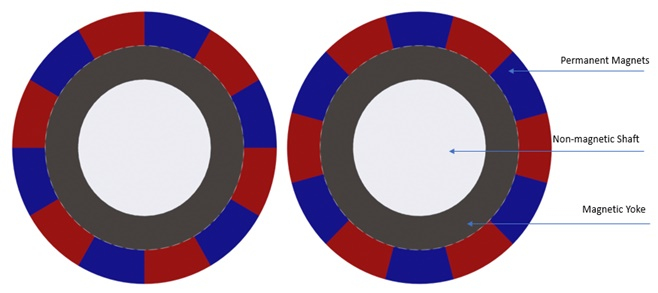Figure 1 - Magnetic gear model with radial magnets

The period of the transmitted torque waveform is 60 degrees calculated using the following formula, 360/n, where n is the number of pole pairs.  Unlike coaxial magnetic gear systems, the torque transmission is realized by the interaction between closest magnets. In other words, only few magnets of the two magnet arrays contribute to the contactless torque transfer. The maximum transmitted torque is reached when the driving gear faces the half of the magnet arc of the driven gear. This can be explained by Figures 2a), 2b) and 2c). They contain plots of the magnetic flux with an overlay of field-lines at the angles of 0, 15 and 45 deg, respectively. In both images, the flux loops illustrate the magnetic field traveling across the air from one gear pole to the second gear pole and goes back through the iron yoke and across the air again. Figure 2a) represents the position when the driving gear set meets the half arc magnet of the driven gear set. At this position, there is a partial overlapping between 3 poles with different magnetizations (2 South poles vs 1 North pole or 2 North poles vs 1 South pole). Therefore, the transmitted force is at its highest value.

Figure 2b) and 2c) show plots of the magnetic field when the gear poles, having same and opposite polarities, are totally facing each other. Hence, the created force is extremely low at these positions.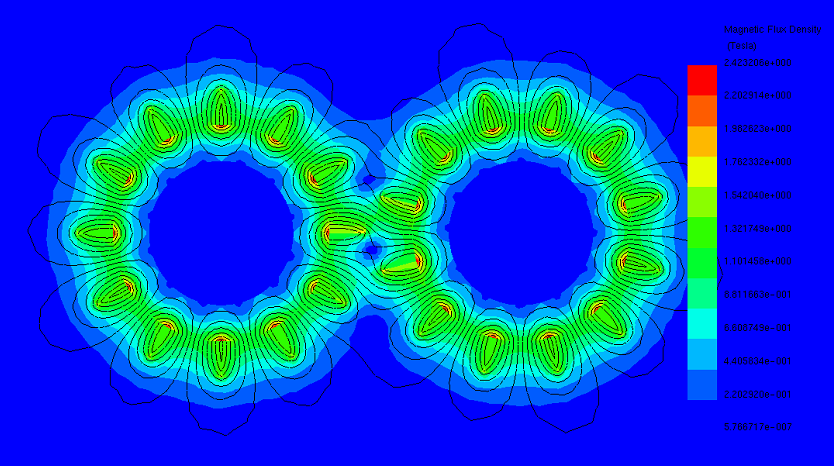(a)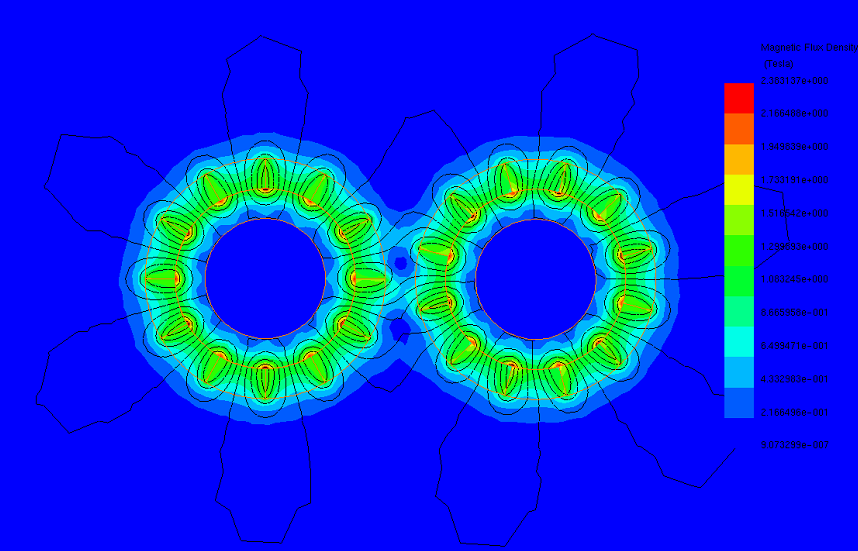(b)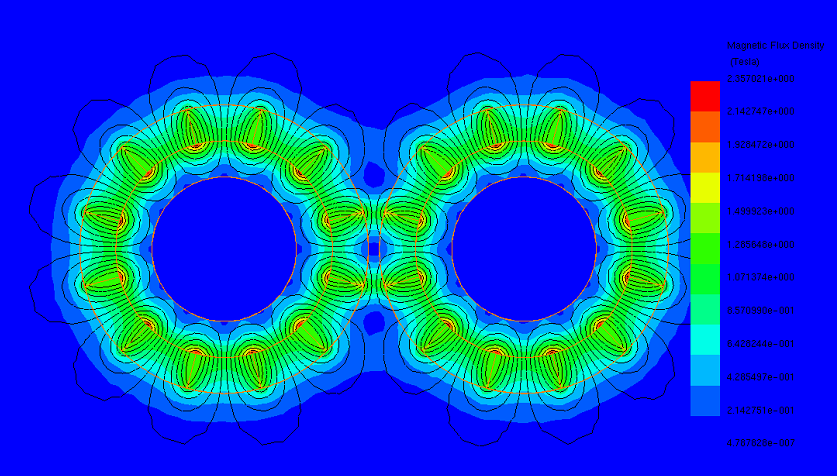(c)

Figure 2 - Magnetic flux density at different position, a) 0 deg, b) 15 deg, c) 45 deg

Figure 3 confirms the interpretations. It shows the torque waveform of the studied external magnetic gear. The torque is computed when the driving gear is rotating while the driven is stationary along one cycle (60 deg). The obtained curve of the torque represents three identical peaks of 1.405 Nm at the angles of 0, 30 and 60 degrees while it crosses the zero at 15 and 45 degrees. Later, both gear sets are moving in opposite direction.

Figure 4 contains the resultant torque in both driving and driven gears. The gear ratio of this system is equal to 1:1. The torque behavior is characterized by few ripples with limited magnitude. The transmitted torque’s average value is 1.395 N.m. Torque ripples can be reduced by increasing the pole pairs.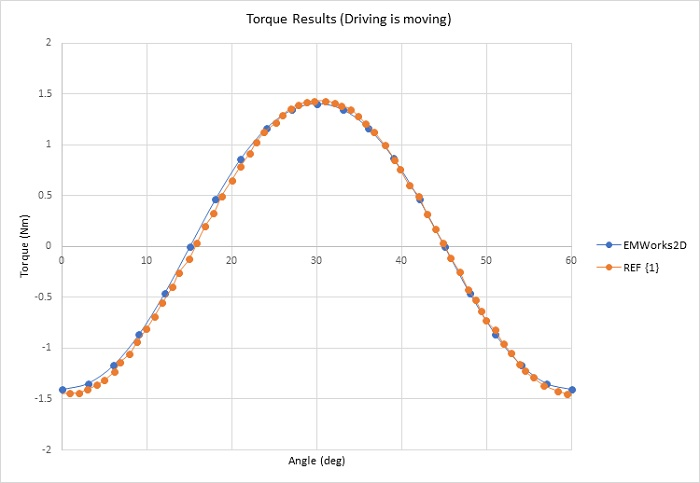Figure 3 - Torque waveform (only driving is moving)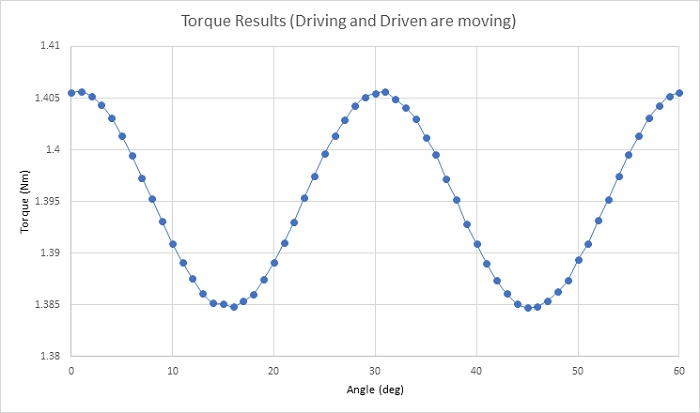Figure 4 - Transmitted torque (both gears are moving)

The maximum transferred torque depends on several factors like number of pole pairs, thickness of the magnets, airgap length, etc. Figure 5a), 5b) and 5c) contain plots of the magnetic flux density at three different airgap lengths from smaller to bigger, respectively. In the Figure 5a), the field is high in the airgap (0.5mm) region. The flux loops between the gear sets show a strong continuity. These loops start to decrease, and the field becomes lower when the airgap is 1.5 mm as shown in the Figure 5b). Figure 5c) demonstrates that most of the magnetic flux is circulating around the poles of the same gear set and does not reach the second gear part because of the high airgap magnetic reluctance. This magnetic reluctance increases with the airgap length.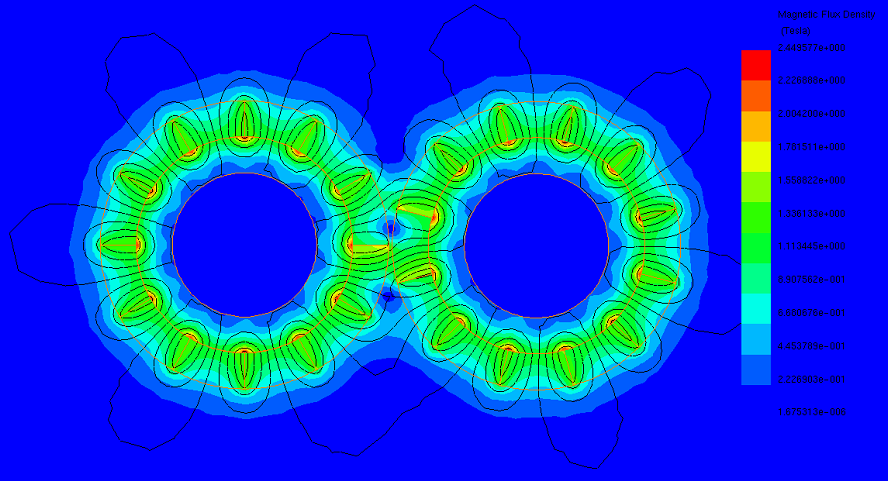(a)(b)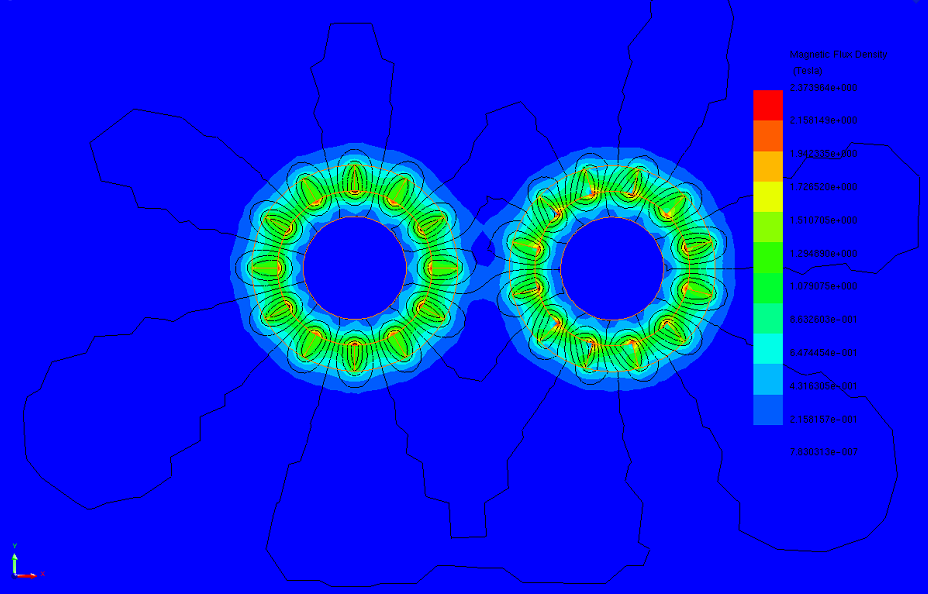(c)

Figure 5 - Magnetic flux results at different airgap, a) 0.5 mm, b) 1.5 mm, c) 5 mm

Figure 6 illustrates the maximum torque values at different airgap distances. It is around 1.40Nm at 1.5 mm while it is around 0.7Nm at 4 mm.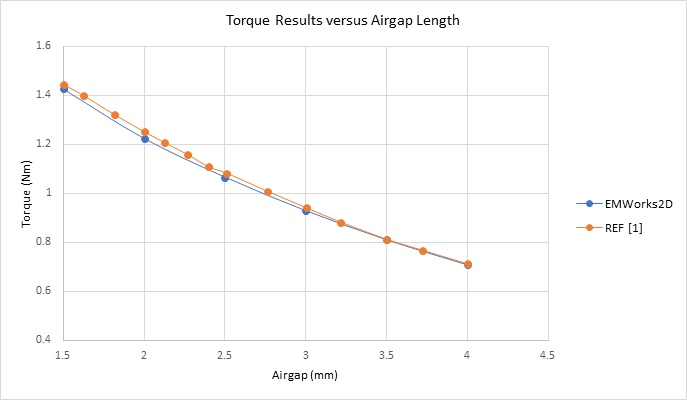Figure 6 - Torque results versus airgap distance

## Example 2: Parallelepiped PM Gear 

The second simulated example, represented in Figure 7, is a magnetic gear model with parallelepiped magnets shape. Each gear set has 6 Neodymium pole pairs with iron yoke. The gear poles can be assumed to have radial magnetization since the magnet’s width is small compared to the gear radius.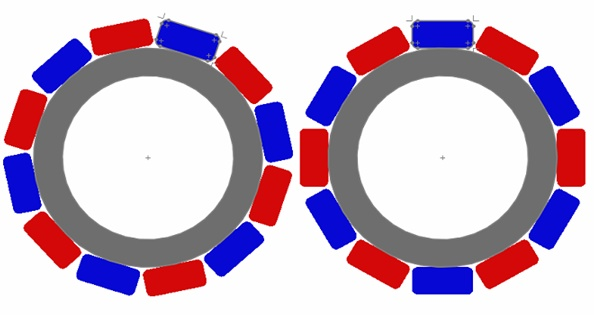Figure 7 - Magnetic gear model with parallelepiped poles

Figures 8a) and 8b) show plots of the magnetic flux density and magnetic vector potential, respectively. These plots are captured when the magnetic gear is at the position of the maximum transmitted torque (15deg). The aligned poles positions are 0 and 30 degrees. Figure 9a) and 9b) represent the magnetic flux and magnetic vector potential plots, consecutively. This position is characterized by opposite coercivity directions of the gear poles. Hence, the torque is null at this position as well as when a driving gear pole meets a full and same polarity driven gear pole.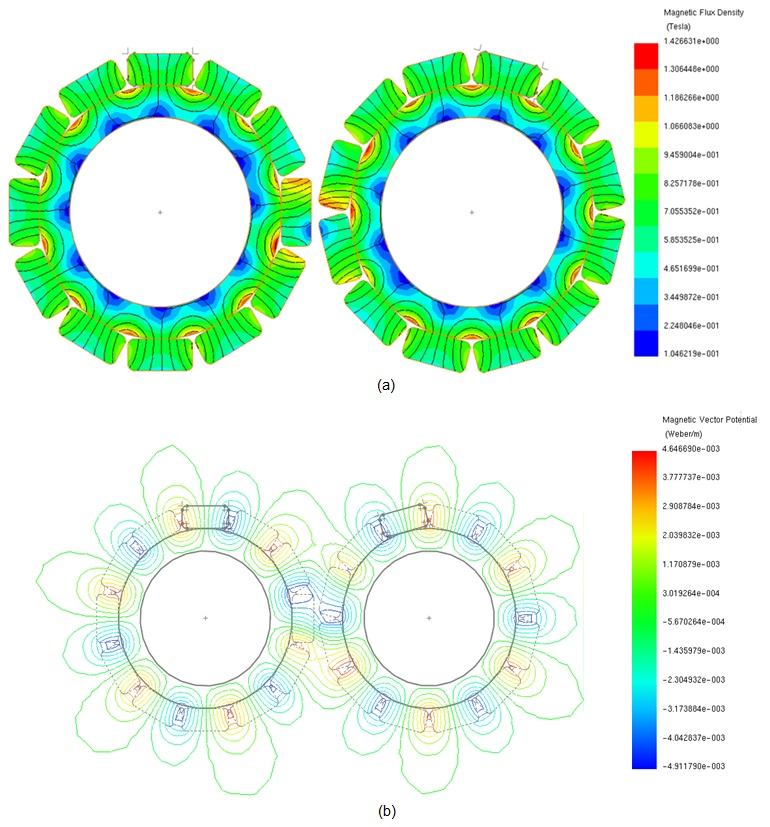Figure 8 - a) Magnetic flux density results, b) Magnetic vector potential results, at 15 deg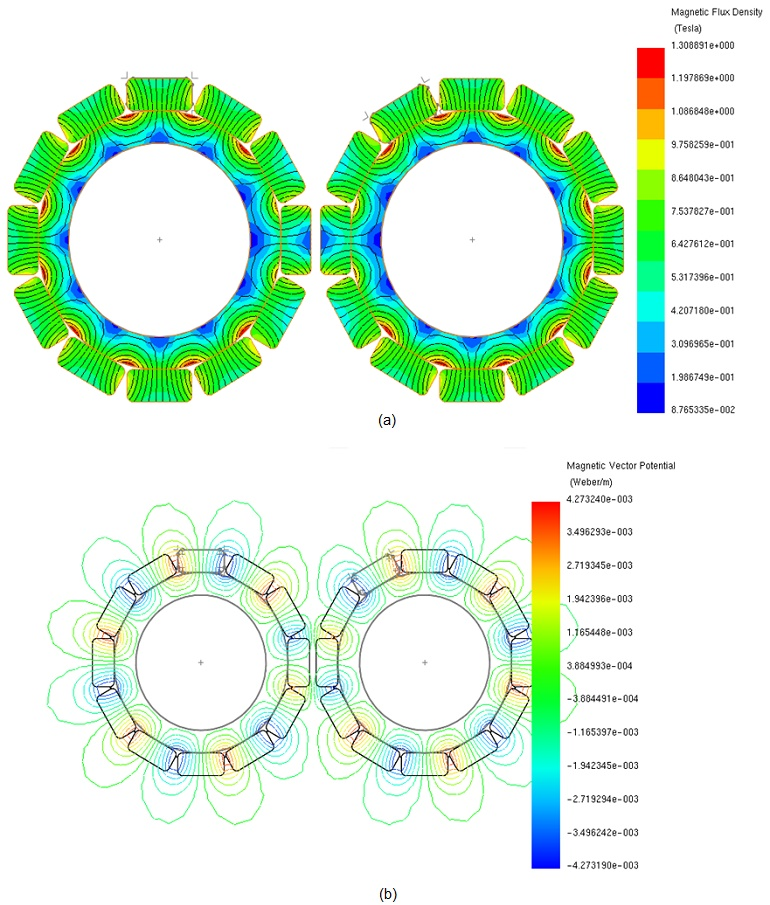Figure 9 - a) Magnetic flux density results, b) Magnetic vector potential results, at 30 deg

The transmitted torque computed when one gear set is rotating and the second is kept steady is shown in Figure 10. The peak value of torque is around 2.8 4Nm and is reached at 15 deg. Figure 11 contains a plot of the torque when both gears are moving. It has a sinusoidal shape with a period of 30 deg.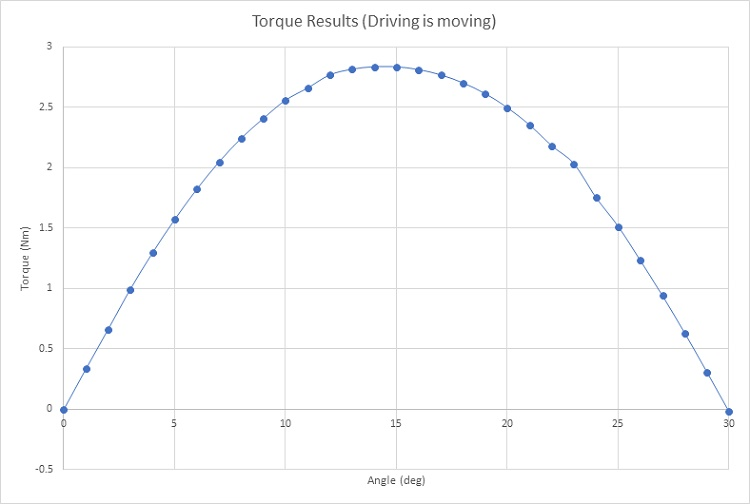Figure 10 - Torque results in case of only the driving is rotating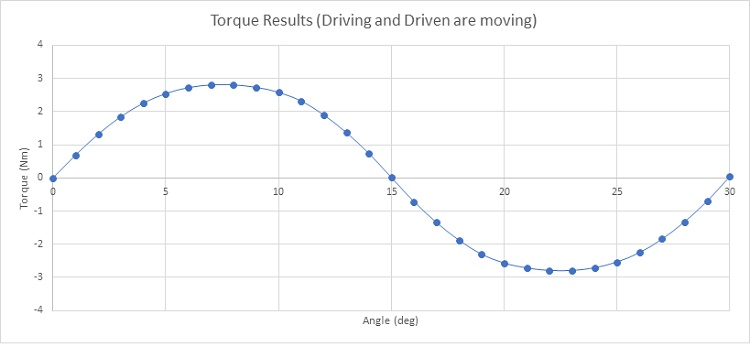Figure 11 - Torque results in case of both driving and driven are rotating

Figure 12 contains torque variation versus airgap length in the case of the magnetic gear model made from 5 and 6 pole pairs. For both configurations, the maximum transmitted torque is achieved at the smallest airgap distance. They are about 2.84Nm and 2.7Nm for magnetic gear with 5 and 6 pole pairs, respectively. Moreover, it can be concluded that a magnetic gear with 6 pole pairs develops higher torque than in case of 5 pole pairs.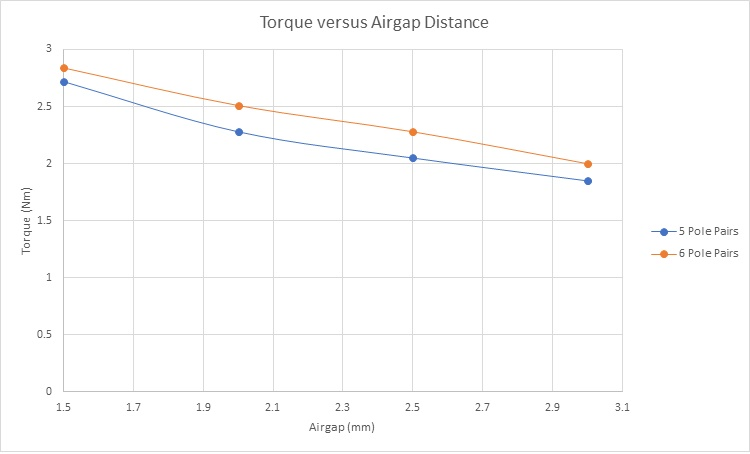Figure 12 - Torque results versus airgap distance

## Conclusion

EMWorks2D was used in this application note to study two different configurations of external magnetic gear. Magnetic flux and torque results were computed versus both angle and airgap length. An excellent conformity of the results was achieved by EMWORKS 2D software as compared to the references.

#### References

: Yi-Chang Wu and Bo-Syuan Jian. Finite-Element Analysis of the Magnetic Field in a Magnetic Gear. Applied Mechanics and Materials Vols. 479-480 (2014) pp 230-233
Mechanism. 1 Mar. 2015, Applied Mathematics & Information Sciences
: Yi-Chang Wu and Chih-Wen Wang. Transmitted Torque Analysis of a Magnetic Gear Mechanism with Rectangular Magnets. 1 Mar. 2015, Applied Mathematics & Information Sciences